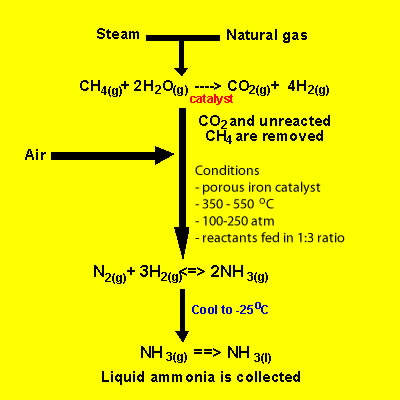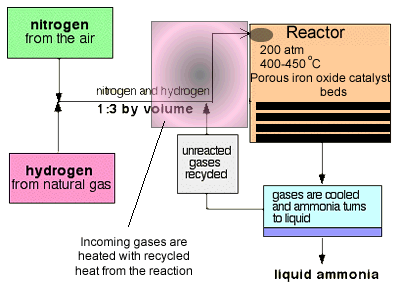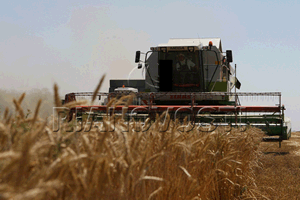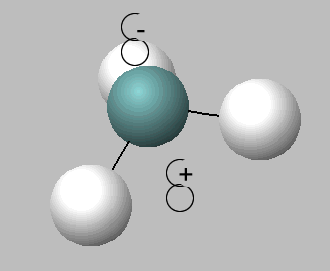Ammonia and the Haber process Not all nitrogen fixation occurs in nature. Nitrogen is reduced to ammonia on an industrial scale in a process called the Haber process. This process uses nitrogen from the air and hydrogen produced from super heated steam and hydrocarbons to form ammonia. A temperature of 300-550oC and a pressure of 100-250 atm in the presence of an iron catalyst provide the conditions necessary to carry out the reaction. Raw material for the formation of ammonia include hydrogen gas, nitrogen gas and hydrocarbons. Hydrogen is obtained, predominantly, from steam reforming of hydrocarbons found in fossil fules. The reaction is summarised below for methane. CH4(g) + H2O(g) => 3H2(g) + CO(g) . This reaction is endothermic and takes place at temperatures that range between 700 and 1100 oC in the presence of a nickel catalyst. A further, slightly exothermic reaction, takes place that produces more hydrogen gas CO(g) + H2O(g) =>H2(g) + CO2(g). The carbon dioxide produced is removed, as is sulfur that might be present in the fossil fuel, prior to ammonia production. Where a cheap supply of electricity is available, as in the hydroelectric generators of Tasmanian electricity supply, electrolysis of water is a possible source of hydrogen. ` `At each pass of the gases through the reactor, only about 15% of the nitrogen and hydrogen converts to ammonia. By continual recycling of the unreacted nitrogen and hydrogen, over several beds of catalyst, to increase the surface area of reaction, the overall conversion is about 98%. The conditions: -The proportions of nitrogen and hydrogen in a 1 to 3 ratio is the right stoichiometric ratio for reaction. Some reactions use excess reactants to increase the yield, according to Le Chatelier's principle, but there is a down side to using excess reactants. Using excess reactants would only limit access of the limiting to the catalyst. You would only use excess reactants in reactions where one of the reactants was expensive and needed to be used up fully. This is not the case here. -The temperature 3H2(g) + N2(g) → 2NH3 (g) (ΔH = –92 kJ/mol) Shifting the position of the equilibrium to the right produces a greater yield of ammonia. According to Le Chatelier's Principle, since the reaction is exothermic, lowering the temperature should drive the system to counteract the change by producing more heat, hence the forward reaction is favoured. However, 400 - 450°C isn't a low temperature! Now at low temperatures the rate of the reaction is slow. From an economic perspective, manufacturers need to produce as much product per hour as possible. There is no use trying to achieve a high yield if it takes several days to do so. The gases stay in contact with the catalyst for a short time so they must reach equilibrium in a very short period of time. The temperature of 400 - 450°C is a compromise between yield and rate. At this temperature the reaction is 15% efficient but occurs at a rate that makes it economically viable. The use of a catalyst does not impact on the final equilibrium yield but increases the rate at which equilibrium is achieved. The pressure will play a significant part in the equilibrium position of the reaction. There are 4 molecules on the left side of the equation below, but only 2 on the right. 3H2(g) + N2(g) → 2NH3(g) According to Le Chatelier's Principle, if pressure is increased the system will respond to counter this by favouring the reaction which produces fewer molecules. That will cause the pressure to fall again. Also at high pressures the rate of the reaction increases as molecules are closer together with a greater chance of colliding and sticking to the catalyst. It is therefore reasonable to assume that manufacturers will go for the highest possible pressure in order to get as much ammonia as possible in the equilibrium mixture. Once more we are confronted by commercial considerations. A pressure of 200 atmospheres is high, but not amazingly high. You see, high pressures need strong pipes, specialised pumps and strong containment vessels that can withstand great forces. This adds to the cost of constructing and maintaining an operational facility.Ammonia is used to make most of the modern day fertilisers for agriculture. This has greatly increased the capacity of soils to produce greater yields of crops. Some of the fertilisers are listed below. The production of fertilisers is an example of ammonia acting as a base. Ammonium sulfate is produced by reacting ammonia with sulfuric acid according to the reaction below.  2NH3(g) + H2SO4(l)=> (NH4)2SO4(s) Ammonium nitrate is produced by reacting ammonia with nitric acid according to the reaction below. NH3(g) + HNO3(aq)=> NH4NO3(s) Ammonium nitrate is also used in explosives Urea is produced by reacting ammonia with carbon dioxide according to the reaction below at 140oC and 100atm pressure. 2NH3(g) + CO2(g)=> NH2CONH2(s) + H2O(l)Ammonia is a very polar molecule. It forms hydrogen bonds between its molecules in the liquid state and interacts with water molecules. Hydrogen bonding makes ammonia highly soluble in water.The combustion of ammonia to nitrogen and water is exothermic 4NH3(g) + 3O2(g) → 2N2 (g) + 6H2O (g) (ΔH = –1267.20 kJ/mol) However if the water produced is in liquid form the energy released is greater, as liquid water has less energy than water vapour so the difference in energy is given off. Like water, ammonia is an amphiprotic substance, it can act as both an acid and a base, hence can undergo self ionisation according to the equation below. 2NH3 (l) <=> NH4+ (aq) + NH2- (aq) (At standard pressure and temperature, [ NH4+ ][NH2- ] = 10-30 M2.) Ammonia can act as a weak reductant as is the case in the Ostwald process. Step 1 Includes the combustion reaction below. Here nitrogen goes form an oxidation state of -3 in NH 3 to +2 in NO. 4 NH3 (g) + 5O2 (g) → 4 NO (g) + 6 H2O (g) (ΔH = −950 kJ/mol) Step 2 Nitric oxide is oxidized again to yield nitrogen dioxide, according to the equation below. Here nitrogen goes form an oxidation state of +2 in NO to +4 in NO2 2 NO (g) + O2 (g) → 2 NO2 (g) (ΔH = −114 kJ/mol) Step 3 This gas is then readily absorbed by water producing nitric acid, according to the equation below. Here nitrogen goes form an oxidation state of +4 in NO2, to +2 in NO and +5 in HNO3 3 NO2 (g) + H2O (l) → 2 HNO3 (aq) + NO (g) (ΔH = −117 kJ/mol) 1) Below are the three steps of the synthesis of nitric acid from ammonia known as the Ostwald process. Give the oxidation states of nitrogen before and after reaction and identify the oxidant and reductant in each. step 1) 4 NH3 (g) + 5O2 (g) → 4 NO (g) + 6 H2O (g) nitrogen changes oxidation state from +3 to +2 -3 to +2 +3 to -2 0 to +2 +4 to +2 and +5 +2 to +4 . The oxidant is ammonia oxygen the reductant is ammonia oxygen step 2) 2 NO (g) + O2 (g) → 2 NO2 (g) nitrogen changes oxidation state from +2 to +4 -3 to +2 +3 to -2 0 to +2 +4 to +2 and +5 +2 to +4 . The oxidant is nitrous oxide oxygen the reductant is nitrous oxide oxygen step 3) 3 NO2 (g) + H2O (l) → 2 HNO3 (aq) + NO (g) nitrogen changes oxidation state from +3 to +2 -3 to +2 +3 to -2 0 to +2 +4 to +2 and +5 +2 to +4 . The oxidant is nitrogen dioxide water the reductant is nitrogen dioxide water 2) 2NH3 (l) <=> NH4+ (aq) + NH2- (aq) This reaction is: an acid / base reaction redox reaction . In this reaction ammonia is acting as an acid only a base only an amphiprotic substance a triprotic acid 3) 2NH3(g) + H2SO4(l)=> (NH4)2SO4(s) This reaction is: an acid / base reaction redox reaction . In this reaction ammonia is acting as an acid only a base only an amphiprotic substance a triprotic acid 4) The Haber process can be summarised by the following equation 3H2(g) + N2(g) → 2NH3(g) (ΔH = –92 kJ/mol) Which of the following conditions will maximise yield? high pressure low pressure pressure has no impact on the yield , high temperature low temperature temperature makes no difference to the yield. , with a catalyst without a catalyst catalyst makes no difference to the yield. 5) High pressure in the Haber process will cause an increase in the rate of reaction cause a decrease in the rate of reaction have no impact on the rate of the reaction and will increase the yield decrease the yield have no impact on the yield. 6) During the Haber process formation of ammonia occurs when reactant molecules are passed over four beds of a porous catalyst. This has the effect of increasing the yield decreasing the yield but increasing the rate increasing the rate but not the yield by increasing the temperature of the reaction increasing the concentration of reactants increasing the surface area of the catalyst 7) Compromises have to be made in industry during chemical synthesis. The greatest yield of ammonia is best achieved by: - high temperatures low temperatures . However, temperatures between 400 and 450 are used because the reactants would liquefy at lower temperatures and the reaction could proceed at an explosive rate. the reaction at lower temperatures is too slow. the yield is too low at lower temperatures. higher temperatures would cause a fire in the reaction chamber. 8) High pressure Low pressure would drive the following reaction 3H2(g) + N2(g) → 2NH3(g) to the right left . In a commercial environment the pressure is kept high kept at a level that is not too high but not too low kept very low kept very high due to financial restraints customer demands for higher ammonia concentrations the synthesis of a more pure product (ammonia) 9) During the Haber process ammonia is constantly removed. Removal of ammonia reduces the pressure and drives the reaction forward. shifts the equilibrium position to the right thus increasing the yield. increases the rate of the reaction by removing the product and allowing the reactant molecules more room to react. 10) Excess reactants are sometimes used to increase the yield. However in this case a volume ratio of nitrogen to hydrogen of 1:3 is used. Why? 11) A 15% efficiency rate is achieved in the Haber process. Although this rate is economically viable, suggest what role temperature plays in such a low rate and why does a catalyst not increase the efficiency? 12) A great deal of heat is produced during the Haber process. What is done with this heat? 13) What conditions would result in the equilibrium below being established most rapidly? high temperature, low pressure low temperature, high pressure high temperature, high pressure . As a result the the conditions would have no impact on the yield. equilibrium yield would be high equilibrium yield would be low. 3H2(g) + N2(g) → 2NH3(g) 14) Ammonia dissolves readily in water. i) Explain why. ii) A solution of ammonia is labeled 0.520 % w/v. Calculate the molarity of this solution to the right number of significant figures is 0.0520 M 0.520 M 0.0306 M 0.371 M 0.52 M 15) Ammonia can be used as a fuel as was the case during WWII when the German military was starved of fossil fuels. 4NH3(g)  +  3O2(g) =>  2N2(g)   +   6H2O(g) (ΔH = –1267.20 kJ/mol) a) If 0.017 g of ammonia was burnt completely in a bomb calorimeter to heat 6.00 g of water at 20oC. What is the final temperature of the water? 2.06 C 32.6 C 24.06 C 18.12 C 29.32 C Solutions Ammonia as a fuel

Home